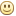Discussion in "Electronics" started by    diana1234    Mar 30, 2015.
Mon Mar 30 2015, 08:12 AM
Dear sir,

How a resistor can be used as a current limiting resistor with negligible voltage drop across it in a circuit.
what criteria to choose a resistor?
please elaborate as i am fresher in electronic design.

Tue Mar 31 2015, 04:29 AM
Its in nature of resistor to reduce the current when connected in series. Voltage drop will always be proportional to current flowing in the circuit. as V = IR
If R is fixed then its the current that cause less or more voltage drop than resistor value as its not changing.

selecting a resistor is according to the final device connected in your circuit. e.g. if you have an LED connected in the circuit say for e.g. like this...
```VCC
---
|    R
+--/\/\/\----|>
|----GND
```

Then selection of R depends on LED characteristics. Now say if LED can sustain a maximum current of 20ma then you have to make sure that resistor should be selected such a way that it limits the current to within 20ma.
V = IR -> R = V/I
If V is 5V and I is 20mA max then:
R = 5 * 1000 / 20 = 250 Ω
You can select any resistor >=250 Ω. As you increase value of resistor from 250 to say 330Ω the current gets limited to 15mA, this will intern reduces the brightness of the LED, and if you keep on increasing resistor the current keeps on reducing at a point when current goes lower than forward bias current of LED. It will stop glowing.

Now we have seen that resistor limits the current but if current flowing from the resistor is high then you have to make sure you select right wattage of resistor.

We know that Power = V * I and I = V/R
so P = V * V / R

Once you have selected the right value of resistor, then required wattage can be calculated from equation above. It is advisable to select a resistor with double wattage that the calculated value.

Hope this clears somewhat your question?
diana1234 like this.
Tue Mar 31 2015, 11:17 AM
Thank you sir for the reply,

As you give the LED example, in that you only disscussed about current, what will be the voltage drop across resistor R (250 ohm, 330 ohm , etc)?

Thank you
Fri Apr 03 2015, 10:34 AM
Voltage drop is always according to current flowing in the circuit.
e.g. If you use 5V and 250Ω resistor then current flowing in the circuit will be
I = V/R = 5/250 = 20mA
so voltage drop across resistor will be V = I * R = 250 * 0.02 = 5V which is reverse of what you did. If you consider a circuit where multiple resistances comes into picture then voltage drop changes. e.g. if you have two series resistors then voltage across each resistor will be:
Vr1 = R1 * V/(R1 + R2)
Vr2 = R2 * V/(R1 + R2)
Sat Apr 04 2015, 04:51 AM
Here is some confusing point
if Vcc = 5volts, I(LED)= 20mA then R=250 ohms as R = V/I.
Also the voltage drop across resistor R is 5volts (V=I*R = 0.02 * 250 = 5 volts)
Now with 5volts voltage drop across resistor R, i think there is no any voltage across LED and LED wont glow at all.
As i know LED requires their minimum rated voltage and current to glow (as LED like a diode then the voltage drop is 0.7 volts minimum).
* Will the LED glow?
* If I use power supply of rating 5Vdc @ 1A then how the Resistor wattage will be calculated, according to load current or supply current if I use formula P = I^2 * R,
if I use P= V^2/R then what is V here, supply voltage or resistor drop voltage?

Please clear these point as I always got stuck at this situation.

Thank you for giving me your valuable time.

[ Edited Sat Apr 04 2015, 05:13 AM ]
Sat Apr 04 2015, 08:16 AM
I was just trying to give an overview of selection criteria and points to take care when selecting a resistor. Ok if you want to go to the scalethen yes led forward voltage do come into picture.
So actual voltage becomes: V-Vled = 5 - 0.7 = 4.3V

So you resistor value becomes R = 4.3 / 20 = 215 Ω
That's the reason most of LEDs use 220 Ω series resistor.

### Information

#### ConversationWed May 12 2021, 03:37 AM
Tanja

### Recent

Mirza123
Thu Jun 25 2020, 03:46 PM
eunicelove124
Mon Jun 22 2020, 03:03 PM
cerouno
Tue Jun 16 2020, 05:10 PM
Marce
Sat Jun 13 2020, 09:43 PM
Davidthils
Thu May 21 2020, 12:44 PM
Jakeror
Thu May 21 2020, 01:28 AM
motorCar
Wed May 20 2020, 07:05 PM
Vordrync
Wed May 20 2020, 11:52 AM

#### Online

Guests: 92, Members: 0 ...

most ever online: 182184
(Members: , Guests: 182184) on 06 Aug 2010: 05:37 AM

Members: 38215